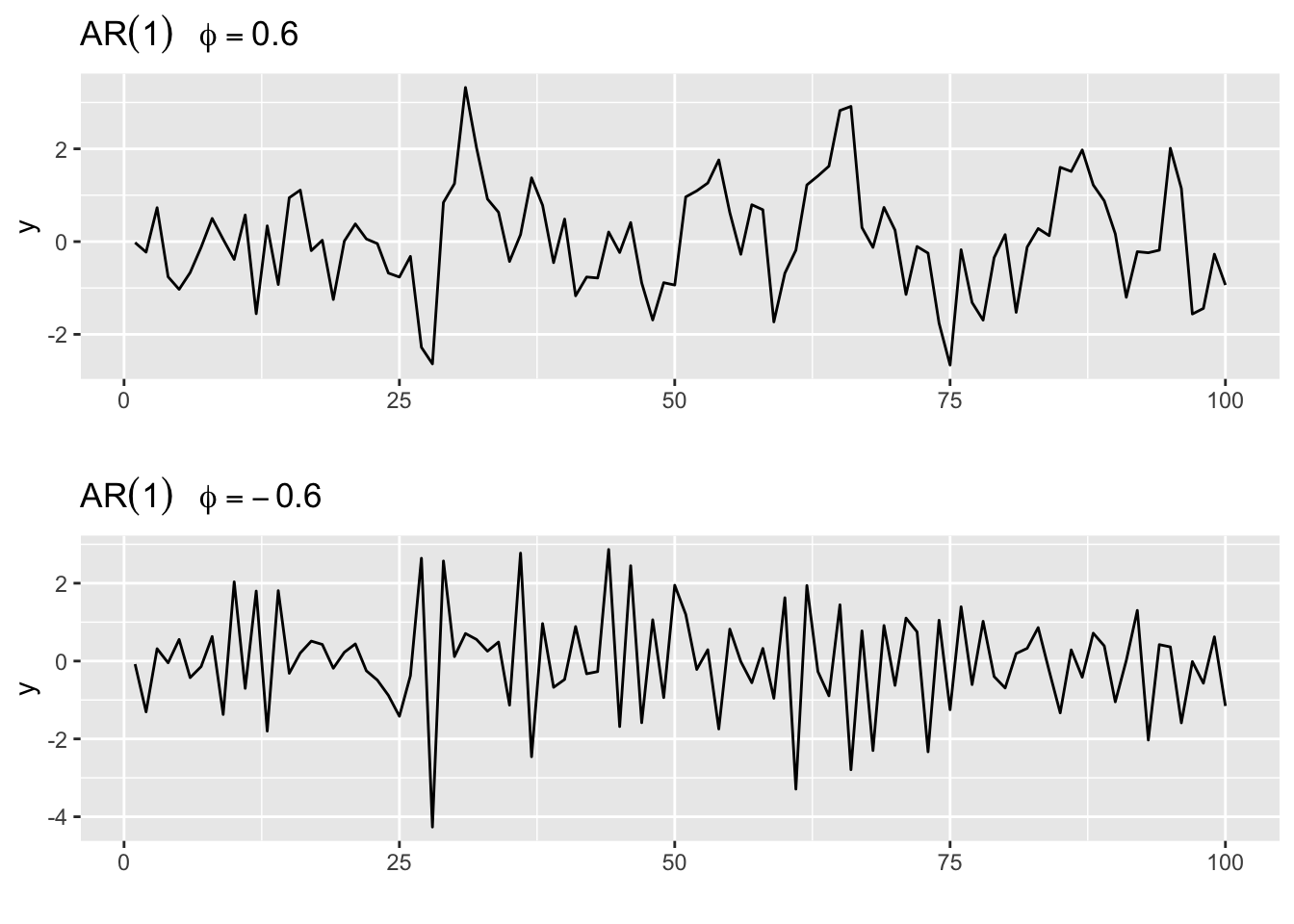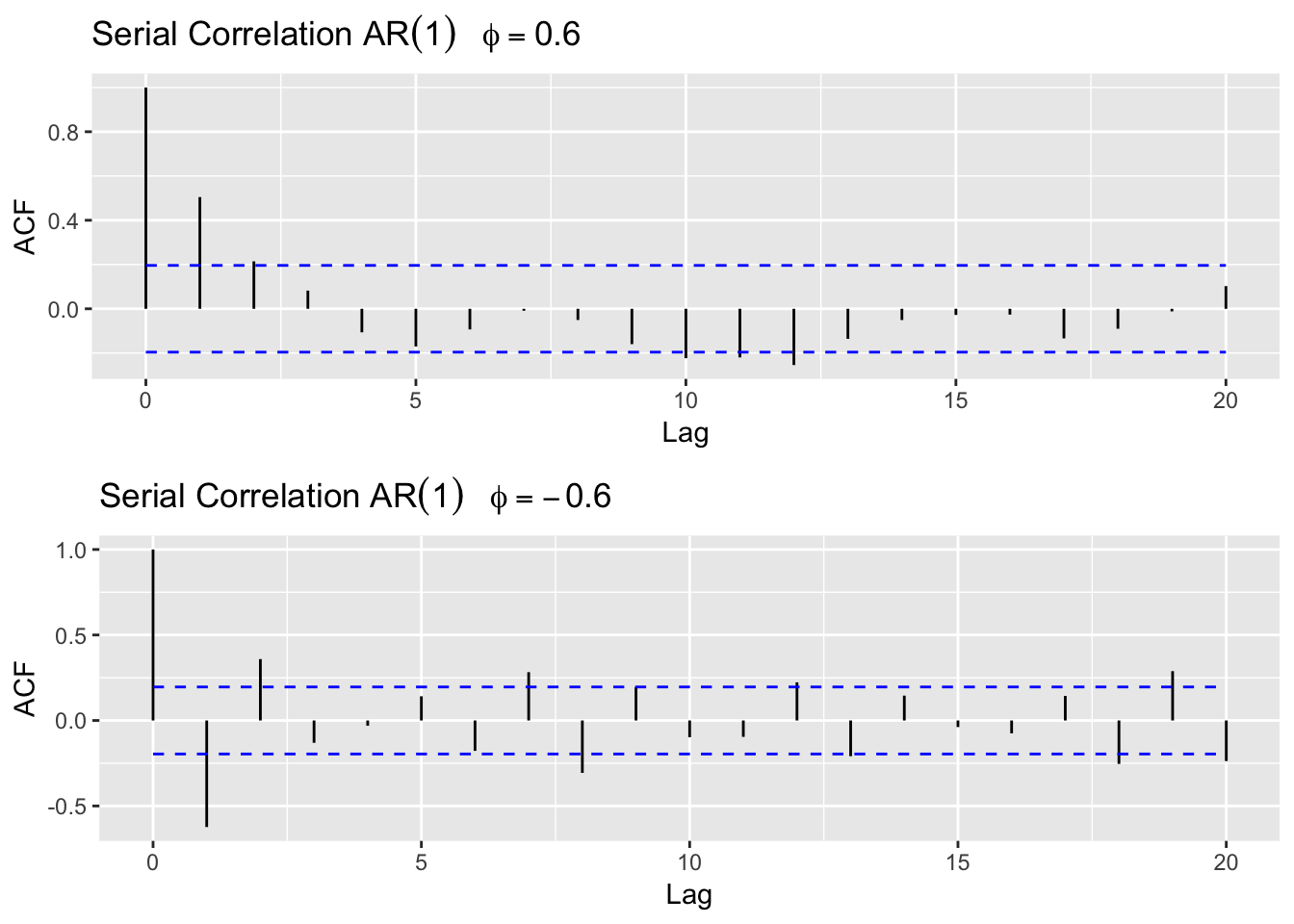The autoregressive process of order $$p$$ is denoted AR($$p$$), and defined by

$y_t = \phi_1y_{t-1}+\phi_2y_{t-2}...+\phi_py_{t-p}+w_t\text{,}$

which can be rewritten as

$y_t = \sum_{j=1}^p\phi_jy_{t-j}+ w_t\text{,}$

where $$\phi_1, \phi_2,..., \phi_p$$ are fixed constants and $$w_t$$ is a random variable mean 0 and variance $$\sigma^2$$.

If the mean, $$\mu$$, of $$y_t$$ is not zero, we replace $$y_t$$ by $$y_t-\mu$$:

$y_t-\mu = \sum_{j=1}^p\phi_j(y_{t-j}-\mu)+ w_t\text{,}$

Autoregressive processes have a natural interpretation as the next value observed is a slight perturbation of the most recent observations, or in other words the current value of the series is linearly dependent upon its previous value, with some random error. This model is called an autoregressive (AR) model, since $$y_t$$ is regressed on itself.

The special case of $$p = 1$$, the first-order process, is also known as Markov process.

We generate two AR(1) series of the form

$$y_t = \phi_1 y_{t-1}+w_t\text{,}$$

where once $$\phi_1 = 0.6$$ and the other time $$\phi_1 = -0.6$$.

set.seed(250)
ar1.1 <- arima.sim(list(order = c(1,0,0), ar = 0.6), n = 100)
ar1.2 <- arima.sim(list(order = c(1,0,0), ar = -0.6), n = 100)

We plot the two series using the autolplot() function.

library(ggfortify)
library(gridExtra)

p1 <- autoplot(ar1.1,
ylab = "y",
main = (expression(AR(1)~~~phi==0.6)))

p2 <- autoplot(ar1.2,
ylab = "y",
main = (expression(AR(1)~~~phi==-0.6)))

grid.arrange(p1, p2, ncol = 1)For an AR(1) model the current value $$y_t$$ of a time series is a function of the past value of the series. We may review the correlational structure by plotting the correlogram.

p1 <-  autoplot(acf(ar1.1, plot = F)) +
ggtitle(expression('Serial Correlation ' *AR(1)~~~phi== 0.6))
p2 <- autoplot(acf(ar1.2, plot = F)) +
ggtitle(expression('Serial Correlation ' *AR(1)~~~phi==-0.6))
grid.arrange(p1, p2, ncol = 1)The autocovariance function for the AR series decays exponentially (for $$\phi >0$$), with a possible sinusoidal variation superimposed (for $$\phi <0$$). Thus, the correlation drops off faster the larger the value of $$k$$.

For an AR(2) model, which can be written as

$$y_t = \phi_1 y_{t-1}+\phi_2 y_{t-2}+w_t\text{,}$$

the current value $$y_t$$ of a time series is a function of the past two values of the series.

Let us generate four different AR(2) series to review their autocorrelational structures.

• AR(2) model I: $$\phi_1 = 0.5$$ and $$\phi_2 = 0.3$$
• AR(2) model II: $$\phi_1 = -0.5$$ and $$\phi_2 = 0.3$$
• AR(2) model III: $$\phi_1 = 1$$ and $$\phi_2 = -0.5$$
• AR(2) model IV: $$\phi_1 = -0.5$$ and $$\phi_2 = -0.3$$.

We make use of the arima.sim() function to generate these four AR(2) models and plot the correlogram by using the autoplot() function in combination with the acf() function. .

ar2.I <- arima.sim(list(order = c(2,0,0),
ar = c(0.5, 0.3)), n = 100)
ar2.II <- arima.sim(list(order = c(2,0,0),
ar = c(-0.5, 0.3)), n = 100)
ar2.III <- arima.sim(list(order = c(2,0,0),
ar = c(1, -0.5)), n = 100)
ar2.IV <- arima.sim(list(order = c(2,0,0),
ar = c(-0.5, -0.3)), n = 100)
p1 <-  autoplot(acf(ar2.I, plot = F, lag.max = 15)) +
ggtitle(expression(AR(2)~~~phi==0.5 * ~~phi==0.3))
p2 <- autoplot(acf(ar2.II, plot = F, lag.max = 15)) +
ggtitle(expression(AR(2)~~~phi==-0.5 * ~~phi==0.3))
p3 <-  autoplot(acf(ar2.III, plot = F, lag.max = 15)) +
ggtitle(expression(AR(2)~~~phi==1 * ~~phi==-0.5))
p4 <- autoplot(acf(ar2.IV, plot = F, lag.max = 15)) +
ggtitle(expression(AR(2)~~~phi==-0.5 * ~~phi==-0.3))
grid.arrange(p1, p3, p2, p4,  ncol = 2)